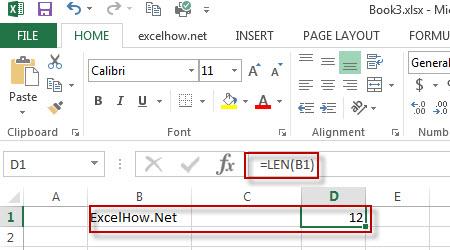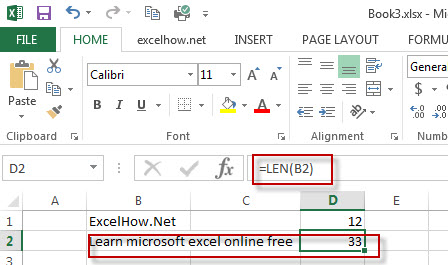# Excel Len Function

This post will guide you how to use Excel LEN function with syntax and examples in Microsoft excel.

### Description

The Excel LEN function returns the length of a text string (the number of characters in a text string).

The LEN function is a build-in function in Microsoft Excel and it is categorized as a Text Function.

The LEFT function is available in Excel 2016, Excel 2013, Excel 2010, Excel 2007, Excel 2003, Excel XP, Excel 2000, Excel 2011 for Mac.

### Syntax

The syntax of the LEN function is as below:

= LEN(text)

Where the LEFT function arguments are:
Text -This is a required argument. The text string that you want to get the length of.

### Example

The below examples will show you how to use Excel LEN Text function to get the length of a text string.

#1 To get the number of characters in the B1 cell, just using formula: =LEN(B1).#2 To get the Length of the text string in B2 Cell, just using formula:=LEN(B2)### More Excel Len Function Examples

• Count Number of Cells with String Length greater than X
You can create a new formula based on the SUMPRODUCT function, the N function and the LEN function to check the string length of each cell and also get the total number that the string length is greater than number 10.……
• Split Text and Numbers
If you want to split text and numbers from one cell into two different cells, you can use a formula based on the FIND function, the LEFT function or the RIGHT function and the MIN function. .…
• Sort Cells by Specific word or words
how to sort cells or text values in a column based on a specific word even if the word is in the text string in the cell. How do I sort cells in a column by the number of a specific word or words in excel……
• Sort List of Cells by Word Count
If you want to sort the list by word count in excel, you need to create an excel formula based on the LEN function and the SUBSTITUTE function…….
• Replace Last Comma in String with “and” word
If you have a list of data (B1:B4) that contain the text string and each word is separated by comma character. And you want to replace the last comma character with a new word “and”.You need to create a new complex formula based on the SUBSTITUTE function, and the LEN function to achieve the result……..
• Removing Salutation from Name
To remove salutation from names string in excel, you can create a formula based on the RIGHT function, the LEN function and the FIND function……
• Insert The File Path and Filename into Cell
If you want to insert a file path and filename into a cell in your current worksheet, you can use the CELL function to create a formual……..